Courses

# Test: Thermodynamics And Thermochemistry - 3

## 30 Questions MCQ Test Physical Chemistry | Test: Thermodynamics And Thermochemistry - 3

Description
This mock test of Test: Thermodynamics And Thermochemistry - 3 for Chemistry helps you for every Chemistry entrance exam. This contains 30 Multiple Choice Questions for Chemistry Test: Thermodynamics And Thermochemistry - 3 (mcq) to study with solutions a complete question bank. The solved questions answers in this Test: Thermodynamics And Thermochemistry - 3 quiz give you a good mix of easy questions and tough questions. Chemistry students definitely take this Test: Thermodynamics And Thermochemistry - 3 exercise for a better result in the exam. You can find other Test: Thermodynamics And Thermochemistry - 3 extra questions, long questions & short questions for Chemistry on EduRev as well by searching above.
QUESTION: 1

Solution:
QUESTION: 2

### dU for Isothermal Process for Vander Waals Gas and Ideal Gas respectively are:

Solution:

Explanation : a) U = (v,T)

dU = (du/dv)T dv + (dU/dT) dT

here, (dU/dT)v = nCv    and    (dU/dV)T = an2/v2

for isothermal process dT = 0

dU = an2/v2 dv

Integrating it,

∫(U2 to U1)dU = ∫(V2 to V1)an2/v2 dv

(U2 - U1) = an2[-1/v](V2 to V1)

ΔU = -an2[1/v2 - 1/v1]

b)we can express the relationship between internal energy and temperature as:

ΔU = CvdT

internal energy is a function of temperature because internal energy of ideal gas comprises of molecular kinetic energy which further depends on the temperature and hence,

For isothermal process, dT = 0, then ΔU = 0

QUESTION: 3

### For an irreversible isothermal expansion of a perfect gas from Vi to Vt, the change in entropy of the gas is:

Solution:
QUESTION: 4

Match the following: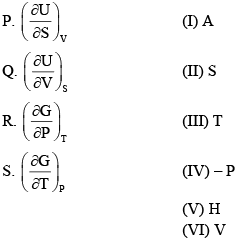Solution:
QUESTION: 5

Standard entropy of crystalline carbon monoxide (in J/mol) at 00K is around:

Solution:
QUESTION: 6

If a gas obeys the equation of state P(V-nb)= nRT, the ratio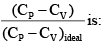Solution:
QUESTION: 7

Two moles of a monoatomic perfect gas initially 4.0 bars and 470C undergoes reversible expansion in an insulated container. The temperature at which the pressure reduces to 3.0 bar is:

Solution:
QUESTION: 8

Choose the correct criterion of spontaneity in terms of the properties of the system alone:

Solution:
QUESTION: 9

For the reaction: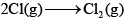The thermodynamic properties:

Solution:
QUESTION: 10

The change in entropy when one mole of an ideal gas is compressed to one-fourth of its initial volume and simultaneously heated to twice its initial temperature is:

Solution:
QUESTION: 11

The internal pressure,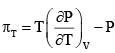for one mole of a Vander Waals gas is:

Solution:
QUESTION: 12

Consider the following P–V diagram for an ideal gas that follows the diagonal path from A to B: The work done (in atm–L) on the gas in the process is: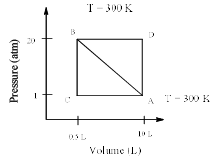Solution:
QUESTION: 13

One mole of an ideal gas (CP = 29.234 JK–1mol–1) is expanded reversibly and adiabatically from 1dm3 to 10 dm3. The initial temperature is 750 K, the final temperature will be:

Solution:
QUESTION: 14

Using Joule–Thompson effect, find the current expression for Δ T:

Solution:
QUESTION: 15

The relationship between volume change in an isothermal process (Δ Vi) and an adiabatic process (Δ Va) for a pressure change from P1 to P2 is:

Solution:
QUESTION: 16

An adiabat ic process is an:

Solution:
QUESTION: 17

For one mole of an ideal gas,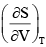is equal to:

Solution:
QUESTION: 18

Which one of the following relations is correct:

Solution:
QUESTION: 19

The change in enthalpy with respect to change in pressure at constant temperature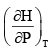is :

Solution:
QUESTION: 20

Find the value of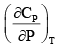for ideal gas:

Solution:
QUESTION: 21

The change in entropy when three moles of argon gas are heated at constant volume from 200 K to 300 K is:

Solution:
QUESTION: 22

For a system of constant composition, the pressure P is given by:

Solution:

du = tds - pdv            at constant entropy ds = 0

so du = -pdv

du/dv=-p

QUESTION: 23

Calculate the coefficient of performance (β) of an engine operating between 1100C and 250C. (The efficiency of Carnot Engine is 22.2%):

Solution:
QUESTION: 24

Δ H and Δ U for the reaction: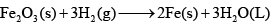At constant temperature are related as:

Solution:
QUESTION: 25

Consider the following statements:

(I) The work done in the reversible isothermal expansion of an ideal gas is greater than that for Vander Waals Gas
(II) When an ideal gas undergoes adiabatic expansion, internal energy increases Which of the statement is true?

Solution:
QUESTION: 26

Match the List-I with List-II and select the correct answers: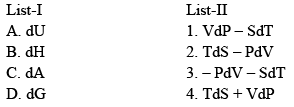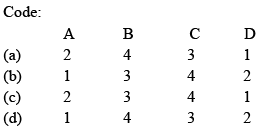Solution:
QUESTION: 27

Which of the following statement is false:

Solution:
QUESTION: 28

One mole of an ideal gas at 300 K is expanded isothermally from an initial volume of 1 L to 10 L. The Δ U for the process is: R = 2 cal/k–mole

Solution:
QUESTION: 29

When 1 mole of a gas is heated at constant volume temperature is raised from 298 K to 308 K. Heat supplied to the gas is 500 J. Then, which statement is correct:

Solution:
QUESTION: 30

Two moles of an ideal gas are expanded isothermally and reversibly from 1 L to 10 L at 300 KJ. The enthalpy change (in KJ) for the process is:

Solution: